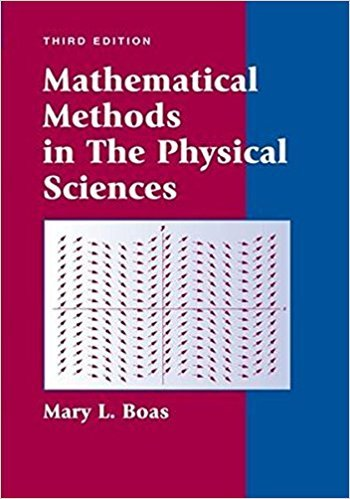×

×

# Solutions for Chapter 5: Multiple Integrals; Applications of Integration## Full solutions for Mathematical Methods in the Physical Sciences | 3rd Edition

ISBN: 9780471198260Solutions for Chapter 5: Multiple Integrals; Applications of Integration

Solutions for Chapter 5
4 5 0 356 Reviews
10
3
##### ISBN: 9780471198260

Since 50 problems in chapter 5: Multiple Integrals; Applications of Integration have been answered, more than 16770 students have viewed full step-by-step solutions from this chapter. Chapter 5: Multiple Integrals; Applications of Integration includes 50 full step-by-step solutions. Mathematical Methods in the Physical Sciences was written by and is associated to the ISBN: 9780471198260. This expansive textbook survival guide covers the following chapters and their solutions. This textbook survival guide was created for the textbook: Mathematical Methods in the Physical Sciences, edition: 3.

Key Physics Terms and definitions covered in this textbook
• //

parallel

• any symbol

average (indicated by a bar over a symbol—e.g., v¯ is average velocity)

• °C

Celsius degree

• °F

Fahrenheit degree